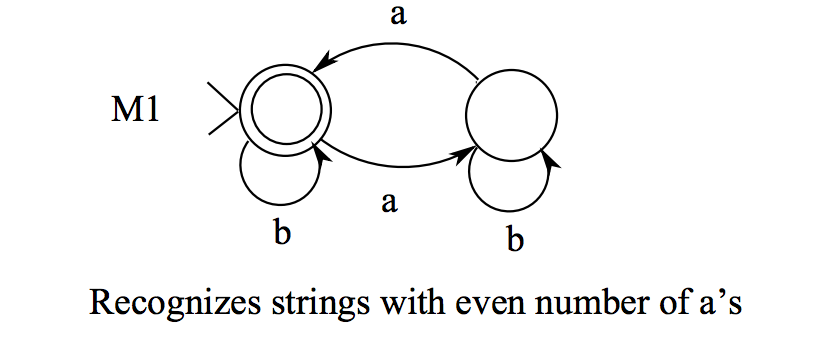```Reflexive is for all x, R has (x,x). all loops are present.
Symmetric is (x,y) in R —> (y,x) All paths are two way.
Transitive is if (x,y) and (y,z) then (x,z). That is, all two step ways I can get in one step.

{a}*{b}* neql {a}*U{b}* because you can’t do aba.

ab (emptyset) = emptyset

Nondeterminstic always guesses right: Because there is at least one computation sequence leading to acceptance the string is accepted by this automaton.

You can take out e arrows without adding states, just add more arrows.

Every n state nondeterminstic without e arrows can be converted to deterministic with at most 2^n states.

If L1 and L2 are regular, then the following are too:
L1 U L2 (union)
L1L2 (concatenation)
L1*
L1 intersect L2

Remember for Kleene star of a sequence, you have to take into account that the starting state has to be accepting. You should have start state excepting then an e arrow to another non accepting state.

Keep in mind￼

If you start with an accepting state, then the regex should include the empty set somewhere/you should star the entire thing because you could do the regex iteratively.

```